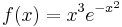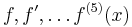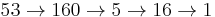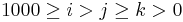# Sage

## Derivatives

2 points Define$f(x)=x^3 e^{-x^2}$ and plot$f, f', \ldots f^{(5)}(x)$ on the interval [ − 2,2]. Use list comprehension for calculating the derivatives and use sum() to add plots into a single picture.

## Collatz

2 points Define a function similar to Collatz:

• If n is odd, let g(n) = 3n + 1
• otherwise divide n by the maximum power of 2 which divides n$2^{a_1}\cdot 3^{a_2}\cdot 5^{a_3} \cdot \ldots \mapsto 3^{a_2}\cdot 5^{a_3} \cdot \ldots$

For example$53 \rightarrow 160 \rightarrow 5 \rightarrow 16 \rightarrow 1$

## Pythagoras

2 points Find all the Pythagorean triples up to 1000. You need$1000\geq i>j\geq k > 0$ where j2 + k2 = i2 and all integers. You cannot list the same triple twice.

## Pythagoras 2

2 points Find all the Pythagorean triples up to 100000. Mind that three for up to 100000 would take days, so you need to generate the triples with a formula, see https://en.wikipedia.org/wiki/Pythagorean_triple#Generating_a_triple